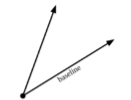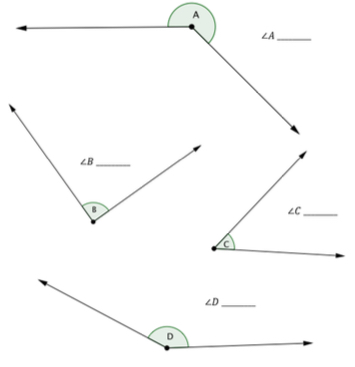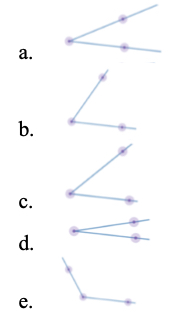# MA.4.GR.1.2Export Print
Estimate angle measures. Using a protractor, measure angles in whole-number degrees and draw angles of specified measure in whole-number degrees. Demonstrate that angle measure is additive.

### Clarifications

Clarification 1: Instruction includes measuring given angles and drawing angles using protractors.
Clarification 2: Instruction includes estimating angle measures using benchmark angles (30°, 45°, 60°, 90° and 180°).
Clarification 3: Instruction focuses on the understanding that angles can be decomposed into non-overlapping angles whose measures sum to the measure of the original angle.

General Information
Subject Area: Mathematics (B.E.S.T.)
Strand: Geometric Reasoning
Status: State Board Approved

## Benchmark Instructional Guide

### Terms from the K-12 Glossary

• Acute Angle
• Angle
• Obtuse Angle
• Right Angle

### Vertical Alignment

Previous Benchmarks

Next Benchmarks

### Purpose and Instructional Strategies

The purpose of this benchmark is to build understanding that angles can be measured. Students have experience identifying acute, obtuse, and right angles (MA.4.GR.1.1). Through instruction in this benchmark, students will attach precise measurements to their informal understanding of the angles they have explored.
• Students will also estimate angle measures based on their growing familiarity of the size of angles according to the benchmark angles 30°, 45°, 60°, 90° and 180°.
• Instruction should allow students to draw angles of all sizes, including situations where they must make angles that are larger than their protractor or their piece of paper. This will ensure that students have an understanding that the angle measure does not change even if the length of the rays do.
• Instruction should use explicit and direct instruction to show students how to use a protractor (standard or circle) to measure and draw angles. Using circle protractors helps students explore reflex angles.
• Instructional time should also be spent breaking apart angles into smaller angles so that students build understanding that angle measures are additive.

### Common Misconceptions or Errors

• Students that have difficulty using a protractor to measure. Assist students with this misconception as they may:
• use the centimeter ruler or inch ruler instead of the baseline when measuring the angles.
• measure the length of each ray and find the sum of the lengths.
• not correctly line up the angle to be measured on the protractor.

### Strategies to Support Tiered Instruction

• Instruction includes using a right angle, 90 degrees measure, as a benchmark to estimate angle measures prior to measuring with a protractor. The teacher provides students with a right angle to overlap with the angles they are measuring as a way to compare their size.
• For example, when given an angle, students will determine if the angle is 90 degrees, greater than 90 degrees, or less than 90 degrees. Students then measure the angle using a protractor and determine if their measurement makes sense based on their estimate.
• The teacher provides angles that have a baseline ray labeled so that students know which ray to line up with the baseline on the protractor and begin their measurement from. Students explain how they will use the protractor to measure the angle (which set of numbers they will use to measure and how they know where to stop measuring).
• For example, the teacher provides an angle similar to the one shown below. Students line up the baseline of the protractor with the ray on the angle that is labeled as the baseline. Students will start measuring with the set of numbers that begins with 0 at the end of the ray and follow the measurements around to the point where the other ray intersects with the protractor.• Use a protractor to find the measure of each indicated angle.### Instructional Items

Instructional Item 1

• Which angles when added together make a right angle?*The strategies, tasks and items included in the B1G-M are examples and should not be considered comprehensive.

## Related Courses

This benchmark is part of these courses.
5012060: Mathematics - Grade Four (Specifically in versions: 2014 - 2015, 2015 - 2022, 2022 and beyond (current))
7712050: Access Mathematics Grade 4 (Specifically in versions: 2014 - 2015, 2015 - 2018, 2018 - 2022, 2022 and beyond (current))
5012055: Grade 3 Accelerated Mathematics (Specifically in versions: 2019 - 2022, 2022 and beyond (current))
5012015: Foundational Skills in Mathematics 3-5 (Specifically in versions: 2019 - 2022, 2022 and beyond (current))

## Related Access Points

Alternate version of this benchmark for students with significant cognitive disabilities.
MA.4.GR.1.AP.2: Using a tool with a square angle, identify angles as acute, right or obtuse and construct angles that are acute, right or obtuse.

## Related Resources

Vetted resources educators can use to teach the concepts and skills in this benchmark.

## Formative Assessments

Drawing and Measuring Angles:

Students are asked to draw and measure acute and obtuse angles whose vertices are at the center of a circle.

Type: Formative Assessment

Using a Protractor to Draw Angles:

Students use a protractor to draw angles with a specified measure.

Type: Formative Assessment

Measuring Angles With a Protractor:

Students are asked to use a protractor to determine the measure of four angles.

Type: Formative Assessment

What Is the Measure of the Angle?:

Students are asked to determine an unknown angle measure that is one component of a larger known angle when given the other components.

Type: Formative Assessment

Town of Happyville:

Students are asked to draw specified angles within a circle in the context of a real world problem.

Type: Formative Assessment

This Angle:

Students are given two angles with the same angle measure but with sides of different lengths and asked to explain which component of an angle determines its measure.

Type: Formative Assessment

Lawn Sprinkler:

Students are asked determine angle measures based on how many one-degree turns are made.

Type: Formative Assessment

Determining An Angle’s Measure:

Students are asked to analyze two angles and explain how their measures are determined.

Type: Formative Assessment

## Image/Photograph

Clipart: Geometric Shapes:

In this lesson, you will find clip art and various illustrations of polygons, circles, ellipses, star polygons, and inscribed shapes.

Type: Image/Photograph

## Lesson Plans

Edible Angles: Decomposing Angles Into Parts of a Whole:

This lesson is designed to review help students apply their understanding that when an angle is decomposed into parts, the measure of the parts is equal to the whole measure of the original angle.

Type: Lesson Plan

Which Angle is Which?:

This is a fun, hands-on activity designed to help students identify and measure obtuse, acute, right, straight and reflex angles. Students create a manipulative tool in their math journals to help them gain understanding of this concept.

Type: Lesson Plan

What's Your Angle? Measuring and Drawing Angles With Protractors:

In this lesson, students will be able to measure various angle sizes using a protractor. Students will also learn how to use a protractor to draw angles when given a specific degree of measure. Students will also know how to compare and contrast angles of different sizes using math terminology.

Type: Lesson Plan

Protractor Power: How to measure and create angles using a protractor:

Students will learn how to use a protractor to measure and create angles of different sizes. Students will work cooperatively to explore this skill.

Type: Lesson Plan

In this lesson students will be introduced to the protractor and benchmark angles, practice reading angles properly, make estimates on angle degrees, measure angles precisely, and participate in a small group activity using their new skills.

Type: Lesson Plan

Angles for \$500:

This lesson engages students in measuring angles using a protractor.

Type: Lesson Plan

In this lesson, students will use addition or subtraction to find the measure of two adjacent angles and determine the measure of an unknown angle.

Type: Lesson Plan

## Original Student Tutorials

Decompose and compose various angles while exploring clocks and windows in this interactive tutorial.

Note: this tutorial exceeds clarification limits and is meant as enrichment for students to improve their problem-solving skills.

Type: Original Student Tutorial

Map the ROC: Angle Measure:

Learn how to measure angles with a protractor to help get a robot through an obstacle course in this interactive tutorial.

Type: Original Student Tutorial

Dash or Bash: Discovering Angles:

Discover what an angle is by helping to program a robot through an obstacle course in this interactive tutorial.

Type: Original Student Tutorial

## Perspectives Video: Teaching Ideas

Discovering Math Vocabulary in Context:

Unlock an effective teaching strategy for vocabulary instruction in this Teacher Perspectives video for educators.

Type: Perspectives Video: Teaching Idea

Finding Angles on Clocks:

Unlock an effective teaching strategy for identifying angles in this Teacher Perspectives video for educators.

Type: Perspectives Video: Teaching Idea

Estimating Angles:

Unlock an effective teaching strategy for teaching students to estimate angle measurements in this Teacher Perspectives video for educators.

Type: Perspectives Video: Teaching Idea

## Tutorial

Solving for a Part of a Decomposed Angle:

This Khan Academy tutorial video presents the strategy for finding the measure of one of two adjacent angles, when the sum of both and measure of one are known.

Type: Tutorial

## MFAS Formative Assessments

Determining An Angle’s Measure:

Students are asked to analyze two angles and explain how their measures are determined.

Drawing and Measuring Angles:

Students are asked to draw and measure acute and obtuse angles whose vertices are at the center of a circle.

Lawn Sprinkler:

Students are asked determine angle measures based on how many one-degree turns are made.

Measuring Angles With a Protractor:

Students are asked to use a protractor to determine the measure of four angles.

This Angle:

Students are given two angles with the same angle measure but with sides of different lengths and asked to explain which component of an angle determines its measure.

Town of Happyville:

Students are asked to draw specified angles within a circle in the context of a real world problem.

Using a Protractor to Draw Angles:

Students use a protractor to draw angles with a specified measure.

What Is the Measure of the Angle?:

Students are asked to determine an unknown angle measure that is one component of a larger known angle when given the other components.

## Original Student Tutorials Mathematics - Grades K-5

Decompose and compose various angles while exploring clocks and windows in this interactive tutorial.

Note: this tutorial exceeds clarification limits and is meant as enrichment for students to improve their problem-solving skills.

Dash or Bash: Discovering Angles:

Discover what an angle is by helping to program a robot through an obstacle course in this interactive tutorial.

Map the ROC: Angle Measure:

Learn how to measure angles with a protractor to help get a robot through an obstacle course in this interactive tutorial.

## Student Resources

Vetted resources students can use to learn the concepts and skills in this benchmark.

## Original Student Tutorials

Decompose and compose various angles while exploring clocks and windows in this interactive tutorial.

Note: this tutorial exceeds clarification limits and is meant as enrichment for students to improve their problem-solving skills.

Type: Original Student Tutorial

Map the ROC: Angle Measure:

Learn how to measure angles with a protractor to help get a robot through an obstacle course in this interactive tutorial.

Type: Original Student Tutorial

Dash or Bash: Discovering Angles:

Discover what an angle is by helping to program a robot through an obstacle course in this interactive tutorial.

Type: Original Student Tutorial

## Tutorial

Solving for a Part of a Decomposed Angle:

This Khan Academy tutorial video presents the strategy for finding the measure of one of two adjacent angles, when the sum of both and measure of one are known.

Type: Tutorial

## Parent Resources

Vetted resources caregivers can use to help students learn the concepts and skills in this benchmark.

## Image/Photograph

Clipart: Geometric Shapes:

In this lesson, you will find clip art and various illustrations of polygons, circles, ellipses, star polygons, and inscribed shapes.

Type: Image/Photograph